EnchantedLearning.com is a user-supported site.
As a bonus, site members have access to a banner-ad-free version of the site, with print-friendly pages.

 You might also like: Containers Words: Write a Question for Each Answer Color Words: Write a Question for Each Answer Beach Words: Write a Question for Each Answer Animal-Related Words: Write a Question for Each Answer Bird-Related Words: Write a Question for Each Answer Today's featured page: Food Webs and Food Chains: Food Theme Page

 Our subscribers' grade-level estimate for this page: 3rd - 4thGo to more writing activities EnchantedLearning.com Write a Question Given an Answer In these worksheets, the student is given a series of short answers. For each answer, the student writes an appropriate question. To get back to the list of worksheets, use the BACK button or click on the picture. Go to Theme Pages
 All Animals Food Geography Holiday House People, Family, Occupations Plants Time, Seasons Transportation, Vehicles Miscellaneous
 All Worksheets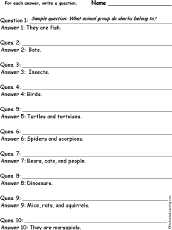Animals: Write a Question for Each Answer In this worksheet, the student is given a series of short answers using animal-related words. For each answer, the student writes a short question.Beach: Write a Question for Each Answer In this worksheet, the student is given a series of short answers using beach-related words. For each answer, the student writes a short question.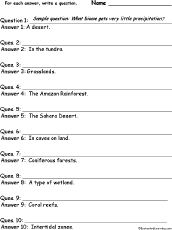Biomes: Write a Question for Each Answer In this worksheet, the student is given a series of short answers using biome-related words. For each answer, the student writes a short question.Birds: Write a Question for Each Answer In this worksheet, the student is given a series of short answers using bird-related words. For each answer, the student writes a short question.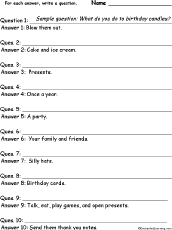Birthday: Write a Question for Each Answer In this worksheet, the student is given a series of short answers using birthday-related words. For each answer, the student writes a short question.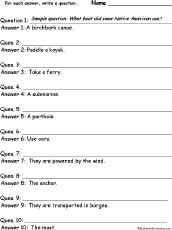Boats and Ships: Write a Question for Each Answer In this worksheet, the student is given a series of short answers using boat-related words. For each answer, the student writes a short question.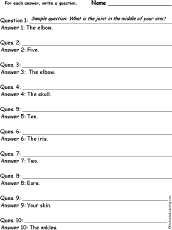Body: Write a Question for Each Answer In this worksheet, the student is given a series of short answers using body-related words. For each answer, the student writes a short question.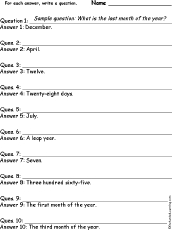Calendar: Write a Question for Each Answer In this worksheet, the student is given a series of short answers using calendar words. For each answer, the student writes a short question.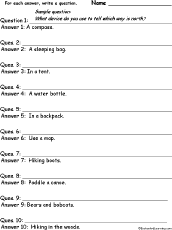Camping: Write a Question for Each Answer In this worksheet, the student is given a series of short answers using camping-related words. For each answer, the student writes a short question.Canada: Write a Question for Each Answer In this worksheet, the student is given a series of short answers using Canadian geography words. For each answer, the student writes a short question. Answers: Arctic Ocean, Pacific Ocean, Atlantic Ocean, Hudson Bay, Baffin Bay, Mount Logan, magnetic North Pole, St. Lawrence River, Mackenzie River, USA.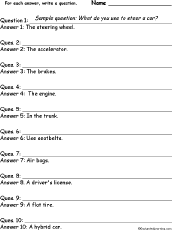Cars: Write a Question for Each Answer In this worksheet, the student is given a series of short answers using car-related words. For each answer, the student writes a short question.Castle and Fairytale: Write a Question for Each Answer In this worksheet, the student is given a series of short answers using castle and fairytale words. For each answer, the student writes a short question.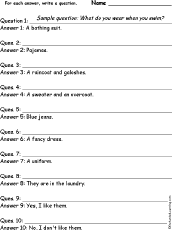Clothes: Write a Question for Each Answer In this worksheet, the student is given a series of short answers using clothing words. For each answer, the student writes a short question.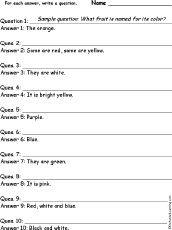Colors: Write a Question for Each Answer In this worksheet, the student is given a series of short answers using color words. For each answer, the student writes a short question.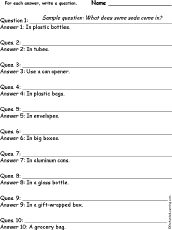Containers: Write a Question for Each Answer In this worksheet, the student is given a series of short answers using container words. For each answer, the student writes a short question.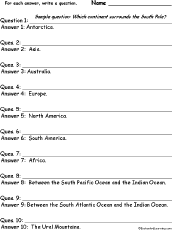Continents: Write a Question for Each Answer In this worksheet, the student is given a series of short answers using continent-related words. For each answer, the student writes a short question.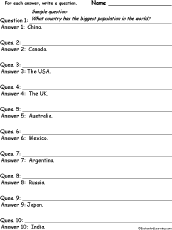Countries #1: Write a Question for Each Answer In this worksheet, the student is given a series of short answers using country names. For each answer, the student writes a short question. Countries: China, Canada, the USA, the UK, Australia, Mexico, Argentina, Russia, Japan, India.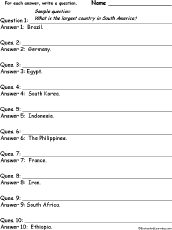Countries #2: Write a Question for Each Answer In this worksheet, the student is given a series of short answers using country names. For each answer, the student writes a short question. Countries: Brazil, Germany, Egypt, South Korea, Indonesia, the Philippines, France, Iran, South Africa, Ethiopia.Countries #3: Write a Question for Each Answer In this worksheet, the student is given a series of short answers using country names. For each answer, the student writes a short question. Countries: Thailand, Mongolia, Somalia, Iceland, Myanmar, Sweden, Nigeria, Turkey, Cuba, Chile.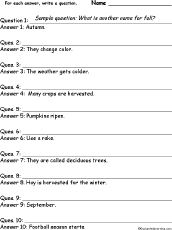Fall: Write a Question for Each Answer In this worksheet, the student is given a series of short answers using autumn-related words. For each answer, the student writes a short question.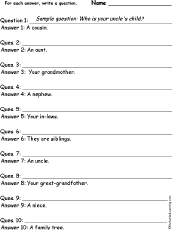Family: Write a Question for Each Answer In this worksheet, the student is given a series of short answers using family-related words. For each answer, the student writes a short question.Farm: Write a Question for Each Answer In this worksheet, the student is given a series of short answers using farm words. For each answer, the student writes a short question.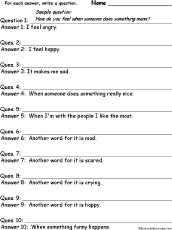Feelings: Write a Question for Each Answer In this worksheet, the student is given a series of short answers using feelings-related words. For each answer, the student writes a short question.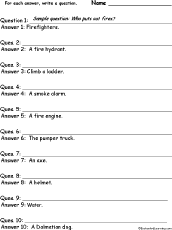Firefighting: Write a Question for Each Answer In this worksheet, the student is given a series of short answers using firefighting words. For each answer, the student writes a short question.Fish: Write a Question for Each Answer In this worksheet, the student is given a series of short answers using fish-related words. For each answer, the student writes a short question.Flowers: Write a Question for Each Answer In this worksheet, the student is given a series of short answers using flower-related words. For each answer, the student writes a short question.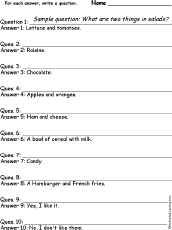Food: Write a Question for Each Answer In this worksheet, the student is given a series of short answers using food words. For each answer, the student writes a short question.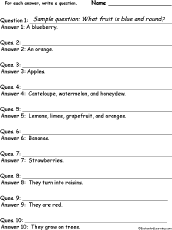Fruit: Write a Question for Each Answer In this worksheet, the student is given a series of short answers using fruit words. For each answer, the student writes a short question.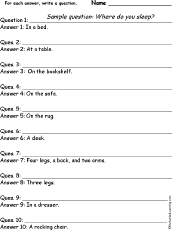Furniture: Write a Question for Each Answer In this worksheet, the student is given a series of short answers using furniture words. For each answer, the student writes a short question.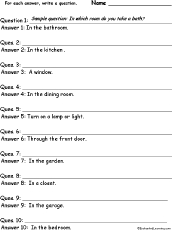House: Write a Question for Each Answer In this worksheet, the student is given a series of short answers using house-related words. For each answer, the student writes a short question.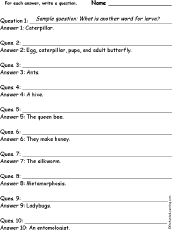Insects: Write a Question for Each Answer In this worksheet, the student is given a series of short answers using insect-related words. For each answer, the student writes a short question.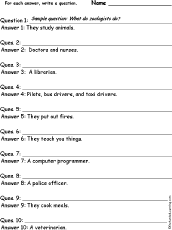Jobs: Write a Question for Each Answer In this worksheet, the student is given a series of short answers using occupation-related words. For each answer, the student writes a short question.US Independence Day - July 4: Write a Question for Each Answer In this worksheet, the student is given a series of short answers using July 4-related words. For each answer, the student writes a short question related to US Independence Day.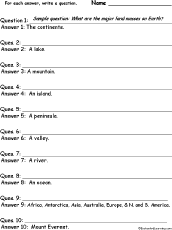Landforms: Write a Question for Each Answer In this worksheet, the student is given a series of short answers using landform-related words. For each answer, the student writes a short question.Metric Measurement: Write a Question for Each Answer In this worksheet, the student is given a series of short answers using metric measurement words. For each answer, the student writes a short question.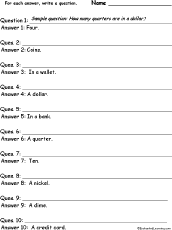Money: Write a Question for Each Answer In this worksheet, the student is given a series of short answers using money-related words. For each answer, the student writes a short question.Oceans: Write a Question for Each Answer In this worksheet, the student is given a series of short answers using ocean-related words. For each answer, the student writes a short question.Parts of Speech: Write a Question for Each Answer In this worksheet, the student is given a series of short answers using parts of speech (nouns, verbs, adjectives, adverbs, etc.). For each answer, the student writes a short question.Pets: Write a Question for Each Answer In this worksheet, the student is given a series of short answers using pet-related words. For each answer, the student writes a short question.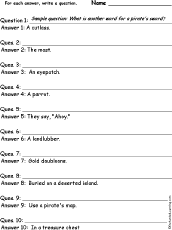Pirates: Write a Question for Each Answer In this worksheet, the student is given a series of short answers using pirate-related words. For each answer, the student writes a short question.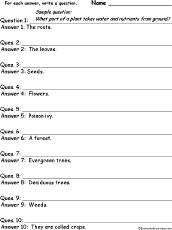Plants: Write a Question for Each Answer In this worksheet, the student is given a series of short answers using plant-related words. For each answer, the student writes a short question.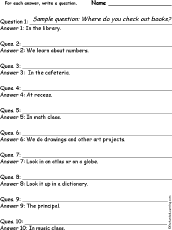School: Write a Question for Each Answer In this worksheet, the student is given a series of short answers using school-related words. For each answer, the student writes a short question.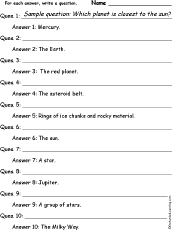Space: Write a Question for Each Answer In this worksheet, the student is given a series of short answers using astronomy words. For each answer, the student writes a short question.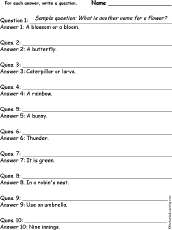Spring: Write a Question for Each Answer In this worksheet, the student is given a series of short answers using Spring words. For each answer, the student writes a short question.Sports: Write a Question for Each Answer In this worksheet, the student is given a series of short answers using sports words. For each answer, the student writes a short question.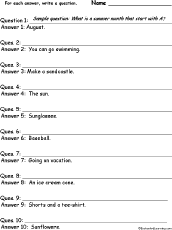Summer: Write a Question for Each Answer In this worksheet, the student is given a series of short answers using Summer words. For each answer, the student writes a short question.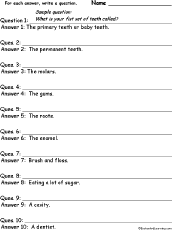Teeth: Write a Question for Each Answer In this worksheet, the student is given a series of short answers using tooth-related words. For each answer, the student writes a short question.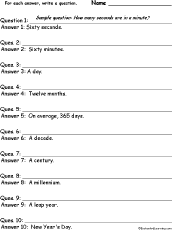Time: Write a Question for Each Answer In this worksheet, the student is given a series of short answers using time words. For each answer, the student writes a short question.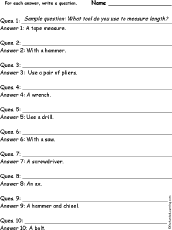Tools: Write a Question for Each Answer In this worksheet, the student is given a series of short answers using tool words. For each answer, the student writes a short question.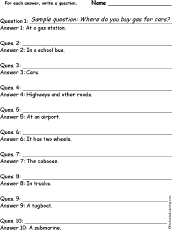Transportation: Write a Question for Each Answer In this worksheet, the student is given a series of short answers using transportation words. For each answer, the student writes a short question.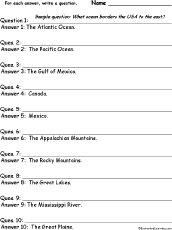US Geography: Write a Question for Each Answer In this worksheet, the student is given a series of short answers about US geography. For each answer, the student writes a short question. Answers: Atlantic Ocean, Pacific Ocean, The Gulf of Mexico, Canada, Mexico, Appalachian Mountains, Rocky Mountains, Great Lakes, Mississippi River, The Great Plains.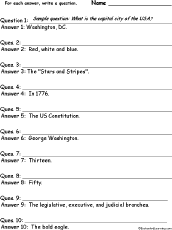US History: Write a Question for Each Answer In this worksheet, the student is given a series of short answers using US history-related words. For each answer, the student writes a short question.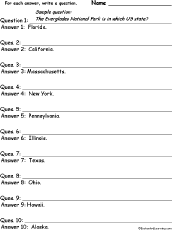US States #1: Write a Question for Each Answer In this worksheet, the student is given a series of short answers using US states. For each answer, the student writes a short question. Answers: Florida, California, Massachusetts, New York, Pennsylvania, Illinois, Texas, Ohio, Hawaii, Alaska.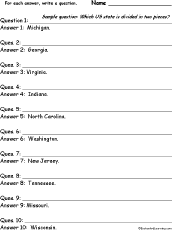US States #2: Write a Question for Each Answer In this worksheet, the student is given a series of short answers using US states. For each answer, the student writes a short question. Answers: Michigan, Georgia, Virginia, Indiana, North Carolina, Washington, New Jersey, Tennessee, Missouri, Wisconsin.US States #3: Write a Question for Each Answer In this worksheet, the student is given a series of short answers using US states. For each answer, the student writes a short question. Answers: Maryland, Arizona, Minnesota, Louisiana, Alabama, Colorado, Kentucky, South Carolina, Oklahoma, Oregon.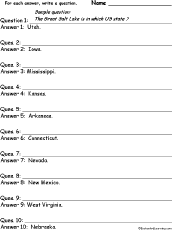US States #4: Write a Question for Each Answer In this worksheet, the student is given a series of short answers using US states. For each answer, the student writes a short question. Answers: Utah, Iowa, Mississippi, Kansas, Arkansas, Connecticut, Nevada, New Mexico, West Virginia, Nebraska.US States #5: Write a Question for Each Answer In this worksheet, the student is given a series of short answers using US states. For each answer, the student writes a short question. Answers: South Dakota, Maine, New Hampshire, Rhode Island, Montana, Wyoming, Delaware, Vermont, Idaho, North Dakota.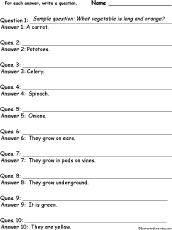Vegetables: Write a Question for Each Answer In this worksheet, the student is given a series of short answers using vegetable words. For each answer, the student writes a short question.Water: Write a Question for Each Answer In this worksheet, the student is given a series of short answers using water-related words. For each answer, the student writes a short question.Weather: Write a Question for Each Answer In this worksheet, the student is given a series of short answers using weather-related words. For each answer, the student writes a short question.Winter: Write a Question for Each Answer In this worksheet, the student is given a series of short answers using winter words. For each answer, the student writes a short question.

All Themes Animals Food People Plants Sports Time and Calendar Holidays
 A B C D E F G H I J K L M N O P Q R S T U V W X Y Z
 A, Long A, Short A All About Me Alligators, Crocodiles Alphabet, Letters Animals Apples April Fool's Day Arbor Day ASL Astronomy B Balloons Baseball Bats Beach Bears Bedtime Bees Birds Birthday Blends Boats Body Bones, Skeletons Butterflies C Calendars Camping Canada Day Categorizing Cats Chickens China Chinese New Year Christmas Cinco de Mayo Circus Classifying Clothing Colors Columbus Day Community Helpers Compare and Contrast Computer Terms Containers Cows D Day of the Dead Dinosaurs Dogs Dolch Words Dolphins Dragons Drawing Ducks Dutch Language E, Long E, Short E Earth Day Easter Eggs Elections Elephants Emancipation Day Emotions Energy F Fact or Opinion Fall Family Farm Father's Day Favorites Feelings Firetrucks, Firefighters Fish Flag Day Flowers Follow Instructions Food French Language Friends Frogs Fruit, Vegetables Furniture G Geography German Language Giraffes Grandparent's Day Groundhog Day H Halloween Hanukkah Happiness Hebrew Holidays Homes/Dwellings Horses Human Body Hundred Days of School I, Long I, Short I Ice Cream Independence Day Insects In The Sky Italian Language J Japan Jobs July 4th Juneteenth K Kangaroo Kings, Queens, Castles Koalas Kwanzaa L Labor Day Ladybugs Leaf Left and Right Letters of the Alphabet M Mammals Martin Luther King Day Memorial Day Mice and Rats Military Money Monkeys Moon Mother's Day Music N Names Newspaper New Year's Day Numbers O, Long O, Short O Oceans/Seas Occupations One Hundred Days of School Opposites Owls P Pandas Penguins People and Community Pets Pigs Pirate Pizza Plants Portuguese President's Day Pumpkins Q R Rabbits Rainbow Reindeer Right and Left Robots Rocks Rodeo Russia S Scarecrows School Seasons Senses Seuss Sewing Shapes Sharks In The Sky Snow Sorting Spanish Language Spelling Spiders Sports Spring Stars Stories St. Patrick's Day Summer Sun Swedish Language Symbols Symmetry Synonyms T Teeth Thanksgiving Time Tools Toys Transportation Travel & Vacation Trees Turkeys U, Long U, Short U V Vacation Valentine's Day Vehicles Veteran's Day W Water Weather Whales, Dolphins Winter Witches, Magic X Y Z

Enchanted Learning®
Over 35,000 Web Pages
Sample Pages for Prospective Subscribers, or click below

 Overview of Site What's New Enchanted Learning Home Monthly Activity Calendar Books to Print Site Index K-3 Crafts K-3 Themes Little ExplorersPicture dictionary PreK/K Activities Rebus Rhymes Stories Writing Cloze Activities Essay Topics Newspaper Writing Activities Parts of Speech Fiction The Test of Time iPhone app TapQuiz Maps - free iPhone Geography Game Biology Animal Printouts Biology Label Printouts Biomes Birds Butterflies Dinosaurs Food Chain Human Anatomy Mammals Plants Rainforests Sharks Whales Physical Sciences: K-12 Astronomy The Earth Geology Hurricanes Landforms Oceans Tsunami Volcano Languages Dutch French German Italian Japanese (Romaji) Portuguese Spanish Swedish Geography/History Explorers Flags Geography Inventors US History Other Topics Art and Artists Calendars College Finder Crafts Graphic Organizers Label Me! Printouts Math Music Word Wheels

## Enchanted Learning Search

 Search the Enchanted Learning website for: